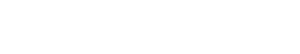# Linear Regression

Linear Regression is a technique used to predict a future outcome based on past data. In the context of text analytics, Linear Regression can be used to predict things like customer sentiment or product popularity.

Linear Regression is similar to other predictive techniques, such as logistic regression or decision trees. However, Linear Regression is unique in that it makes predictions based on a linear relationship between predictor variables and the outcome variable. This means that Linear Regression can be used to predict outcomes even when there is no clear cause-and-effect relationship between the predictor variables and the outcome variable.

## Calculations for Linear Regression

Calculations for Linear Regression are best performed using a software package like R or Python. However, it is possible to perform Linear Regression without any programming knowledge.

There are many online calculators that can be used to perform Linear Regression. Simply enter the data points into the calculator, and the calculator will output the results of the regression.

Linear Regression is a useful tool for text analytics because it can be used to make predictions about future events. However, it is important to remember that Linear Regression is only a tool, and it should not be used to make decisions about future events without first considering other factors that may affect the outcome.

## Linear Regression on Text Data

Linear Regression on text data can be difficult because of the high dimensionality of the data. This means that there are many variables that can potentially influence the outcome variable. For this reason, it is important to use a software package like R or Python to perform Linear Regression, so that you can control for all of the potential predictor variables.

When using Linear Regression on text data, it is also important to remember that the results of the regression should be interpreted in the context of the other variables that were not included in the regression. This is because the results of the regression may be influenced by these other variables.

In conclusion, Linear Regression is a useful tool for text analytics. However, it is important to remember that Linear Regression is only a tool, and it should not be used to make decisions about future events without first considering other factors that may affect the outcome. Additionally, Linear Regression on text data can be difficult because of the high dimensionality of the data. For this reason, it is important to use a software package like R or Python to perform Linear Regression, so that you can control for all of the potential predictor variables.Unlock the power of actionable insights with AI-based natural language processing.